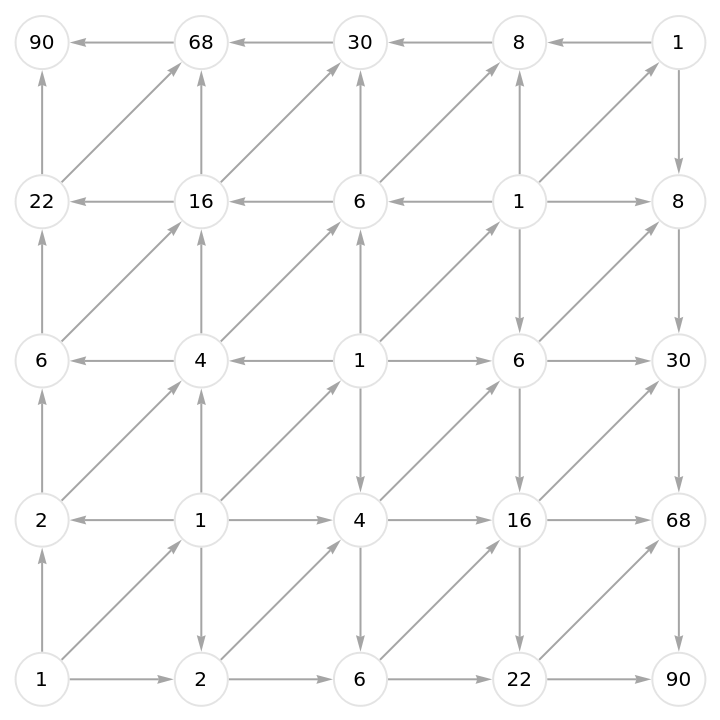Function Repository Resource:

# ToDirectedAcyclicGraph

Convert any undirected graph to a cycle-free directed graph

 ResourceFunction["ToDirectedAcyclicGraph"][g,v0] updates undirected graph g by assigning directions to edges, such that directions point along shortest paths from any one initial vertex v0 to all others. ResourceFunction["ToDirectedAcyclicGraph"][g,{v1,v2,…}] returns a directed acyclic conversion of graph g with directions indicating shortest paths from the nearest initial vertex vi.

## Details

ResourceFunction["ToDirectedAcyclicGraph"] takes at least the same options as Graph.
ResourceFunction["ToDirectedAcyclicGraph"] enables finding shortest paths and counting them.
This is accomplished as follows: First associate every vertex vi with a graph distance di. Then when an edge goes between vertices vi and vj, so long as di<dj, the assigned direction is from vi to vj.
Distance di is defined using GraphDistance as follows:
 for one initial vertex v0 di=GraphDistance[g,v0,vi] for many initial vertices {v1,v2 , …} di=Min[GraphDistance[g,#,vi]&/@{v1,v2,…}]
A difficulty arises with conflicted edges, which join two vertices vi and vj with di==dj.
ResourceFunction["ToDirectedAcyclicGraph"] takes two additional options specifying how to handle conflicted edges.
An optional "CollisionFunction"cfun specifies a SortBy condition for vertices on a conflicted edge so that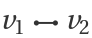is converted to DirectedEdge@@SortBy[{v1,v2},cfun].
Default assignment "CollisionFunction"None passes collision handling to a second conditional.
With "ConflictedEdges"False, ResourceFunction["ToDirectedAcyclicGraph"] deletes conflicted edges.
With "ConflictedEdges"True, ResourceFunction["ToDirectedAcyclicGraph"] returns conflicted edges as undirected edges.

## Examples

### Basic Examples (2)

Determine all shortest paths on a random graph:

 In:=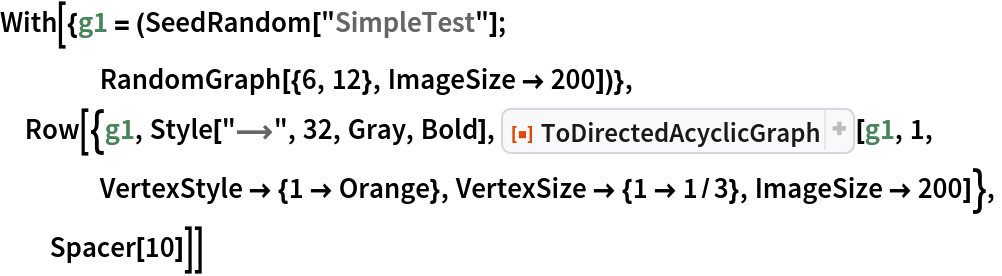Out=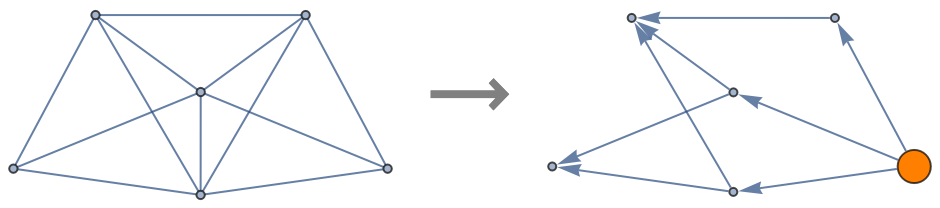Verify that the output above is indeed directed and acyclic:

 In:=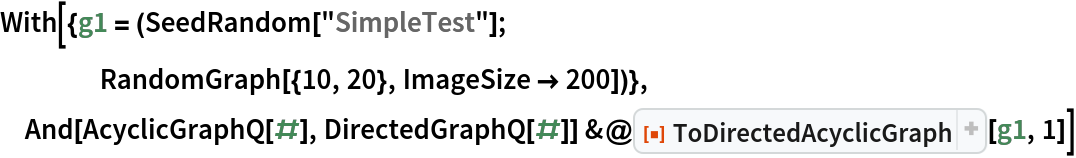Out=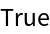### Scope (3)

Explore traversal maps of a Petersen graph:

 In:=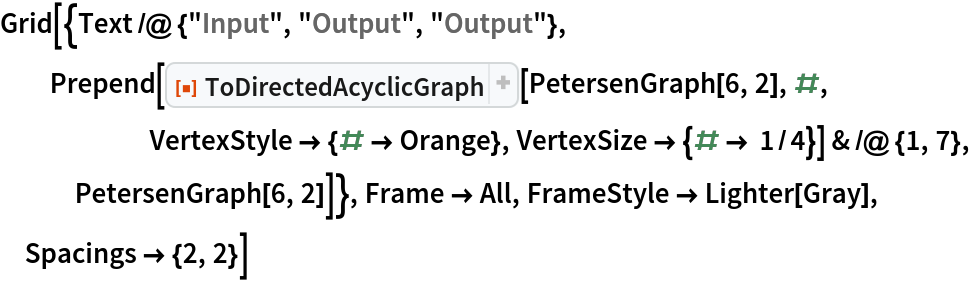Out=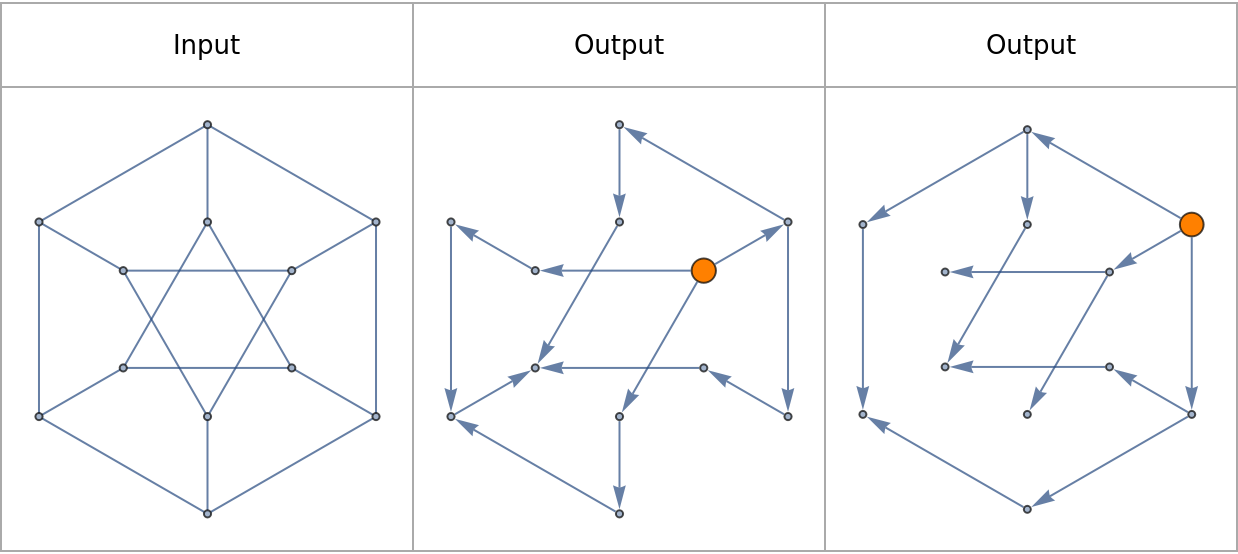Add another Graph-exploring reference point and compare possible pairwise dance moves:

 In:=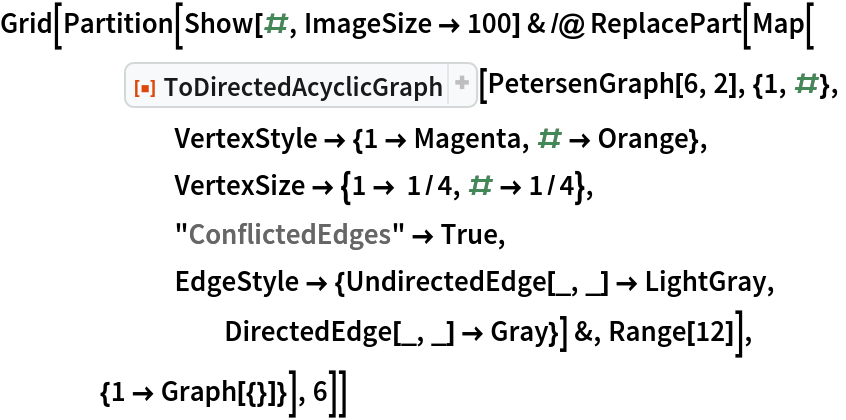Out=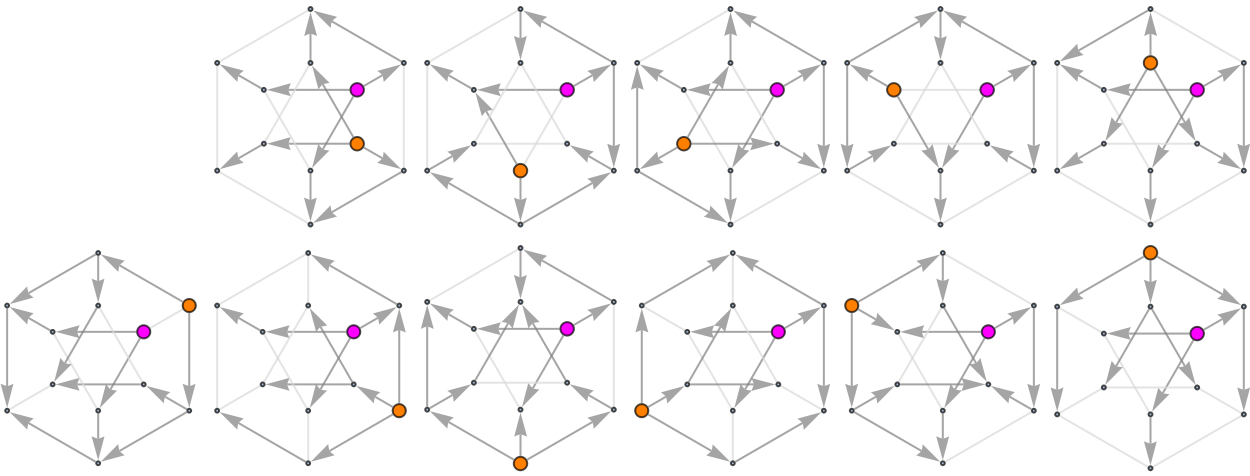Check to see that edges are lost only on odd cycles:

 In:=Out=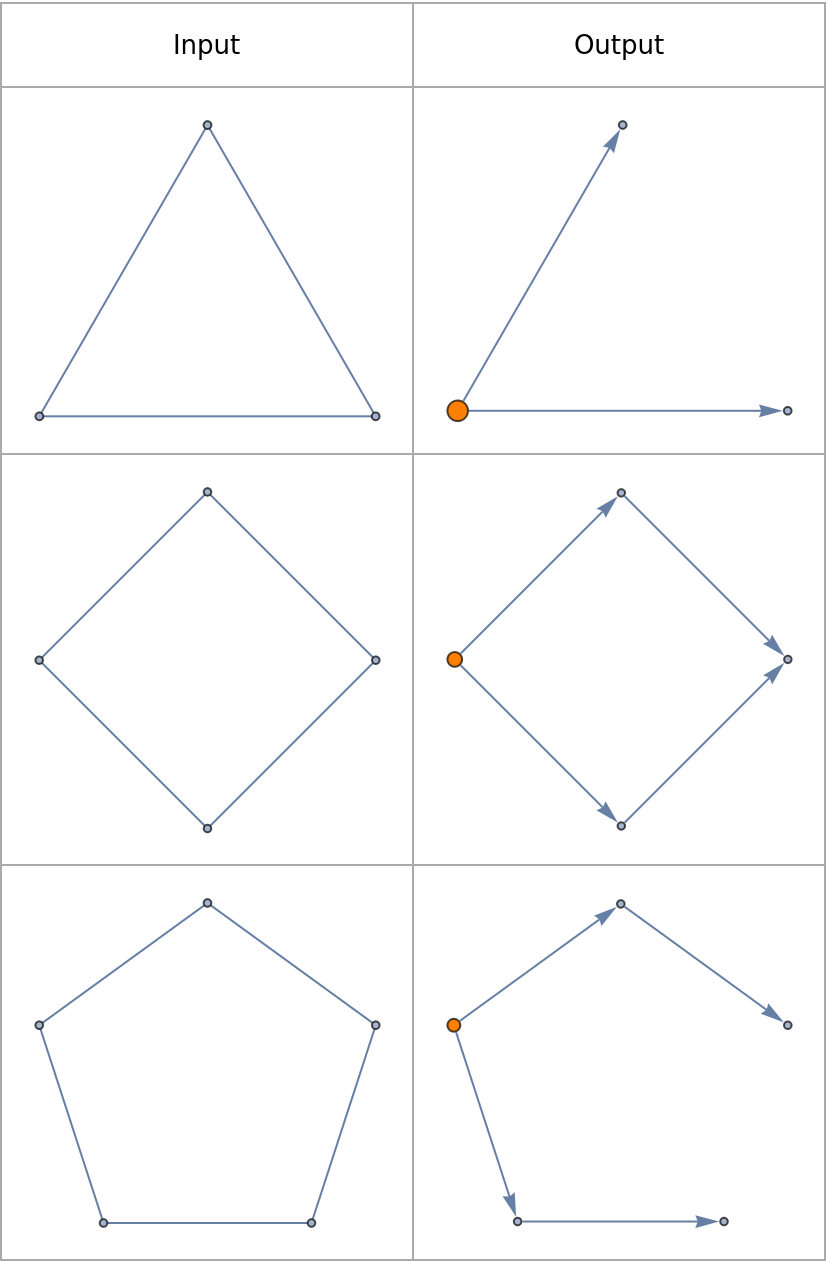Compare what happens when different initial conditions are chosen:

 In:=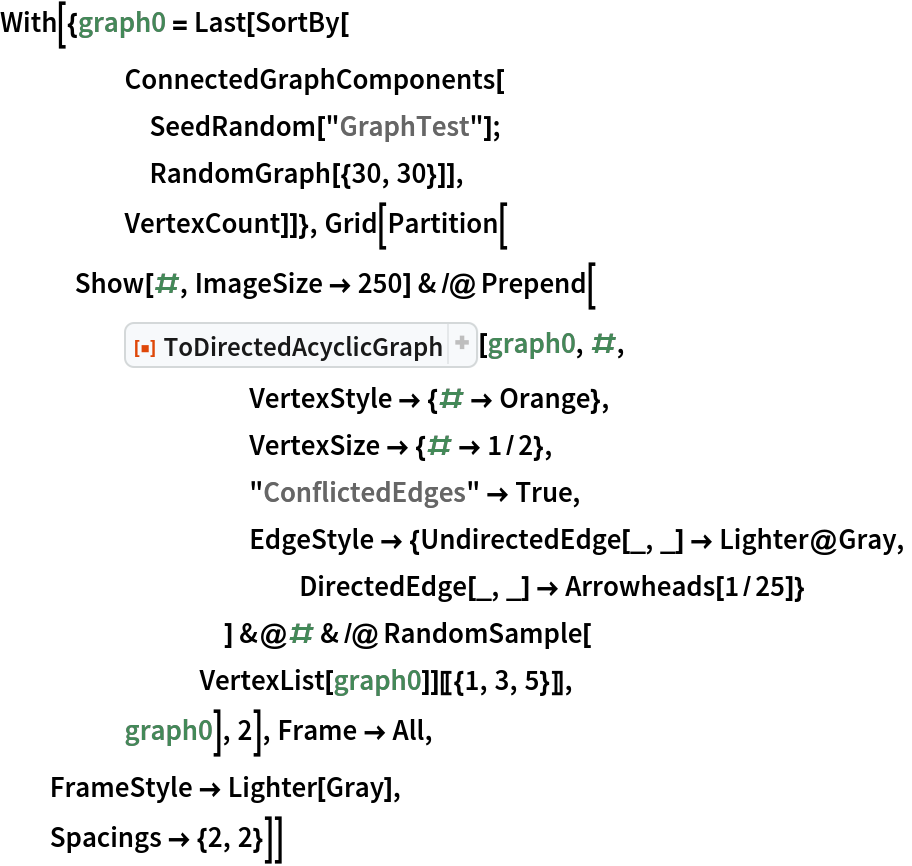Out=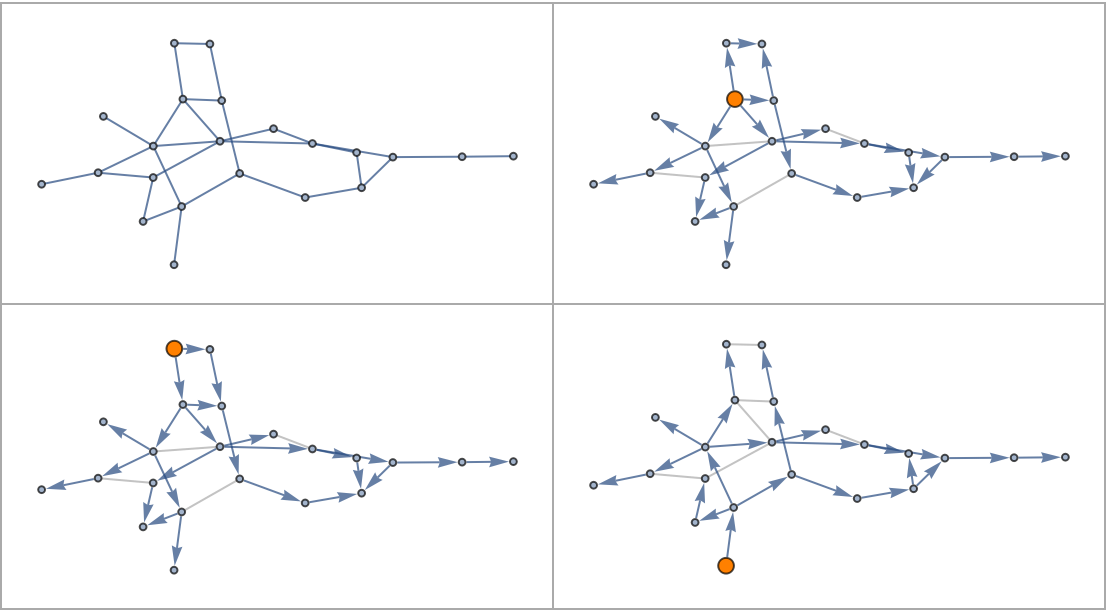### Options (3)

Acyclic directed graphs can be plotted as causal graphs by setting VertexCoordinates to Automatic:

 In:=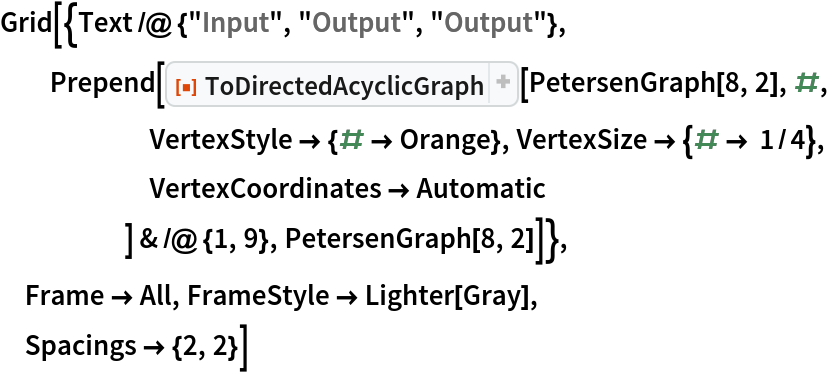Out=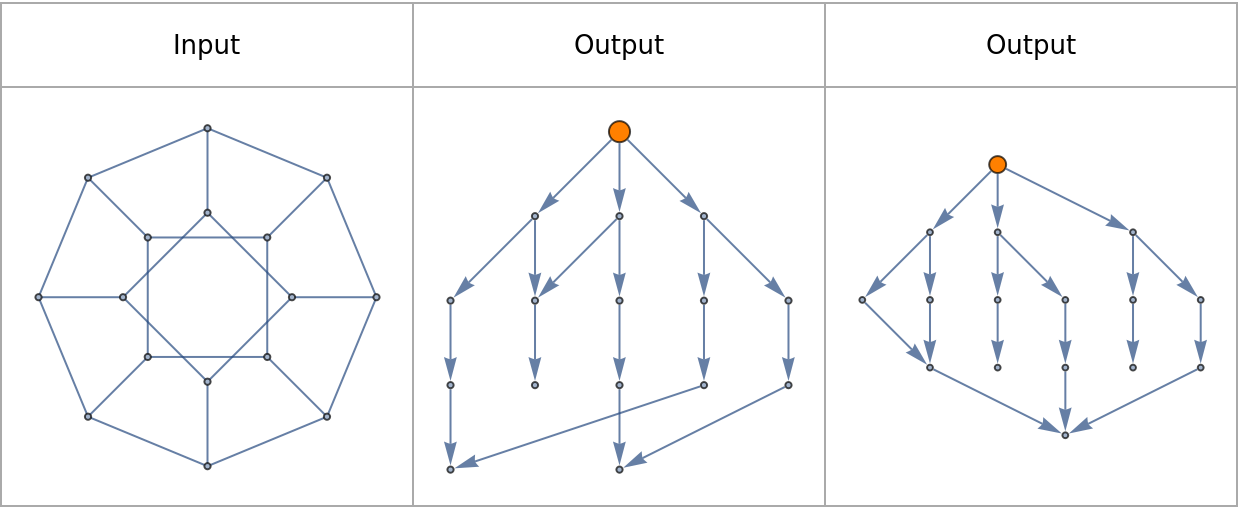Plot a few more causal graphs:

 In:=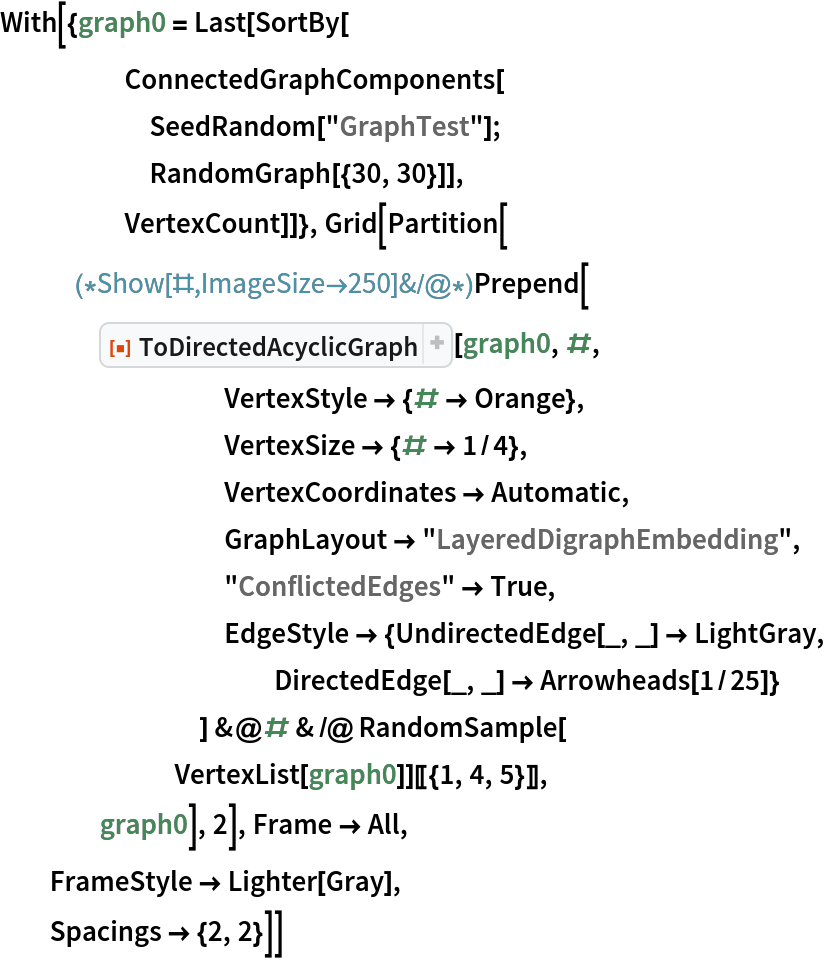Out=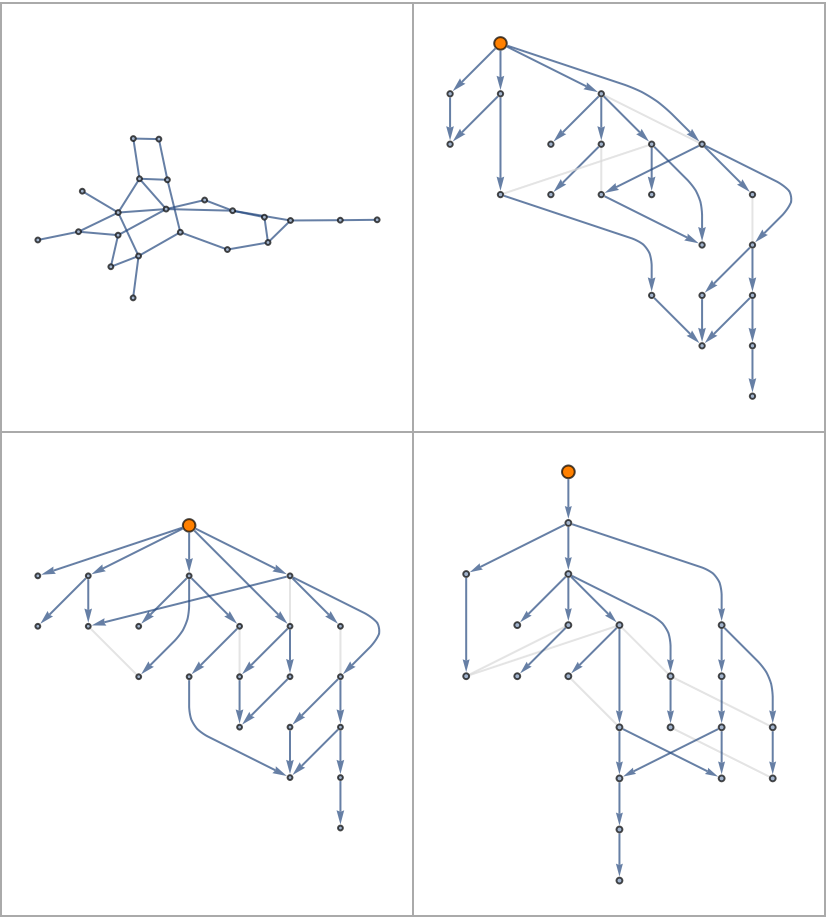Resolve conflicted edges by specifying a CollisionFunction:

 In:=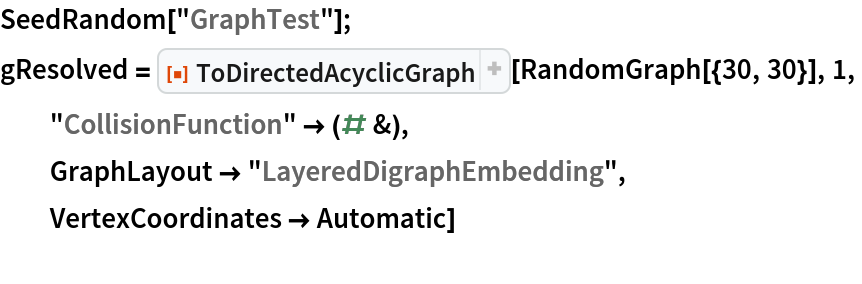Out=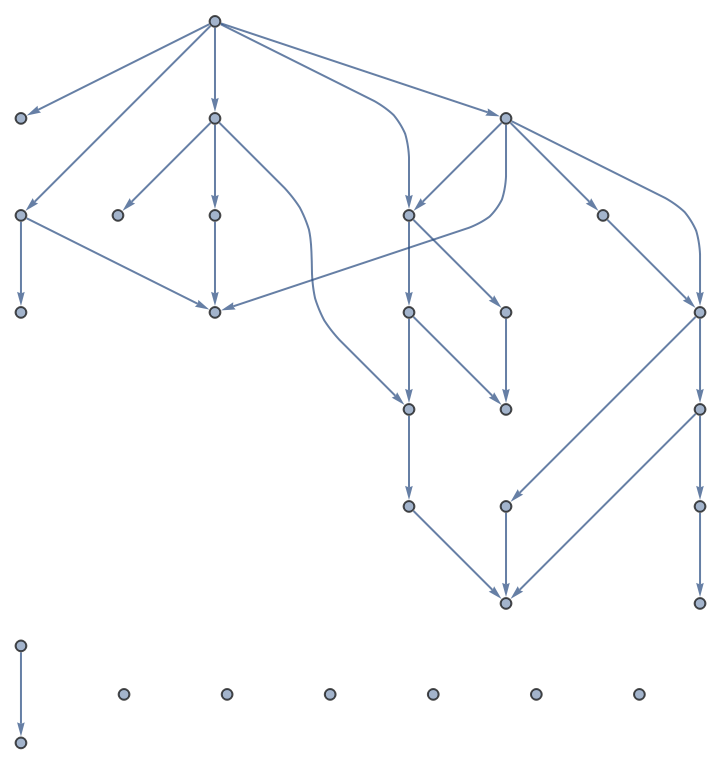Check non-uniqueness of path lengths:

 In:=Out=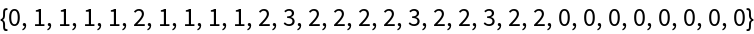### Properties and Relations (2)

The transformation acts as the identity on rows of the GraphDistanceMatrix:

 In:=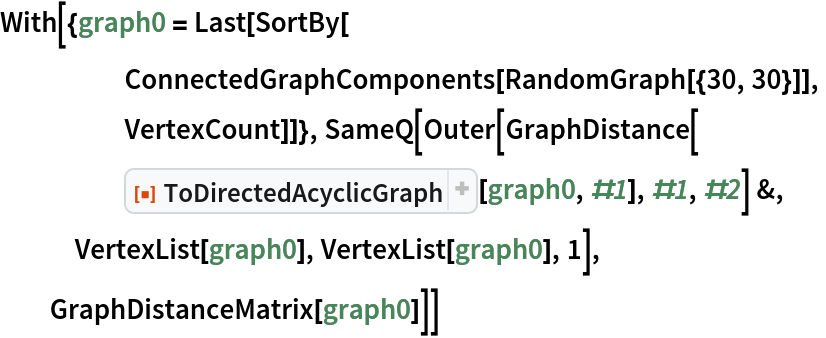Out=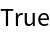Using Identity as the CollisionFunction returns the same output as DirectedGraph with "Acyclic" conversion:

 In:=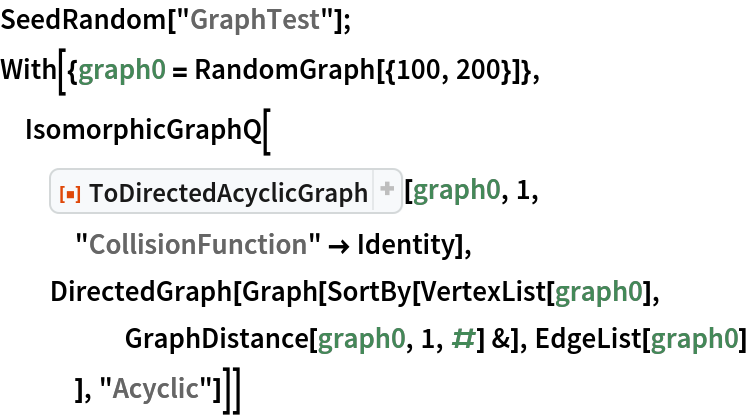Out=### Possible Issues (3)

Specifying multiple reference points can divide the graph into disconnected components:

 In:=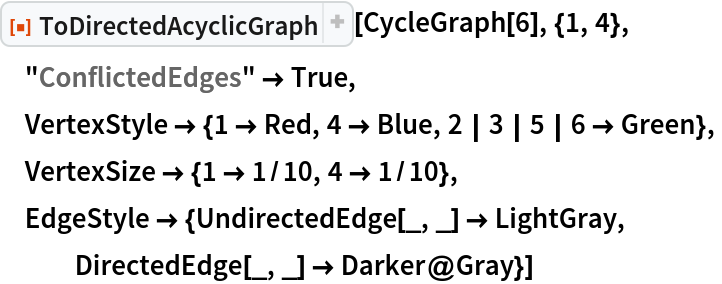Out=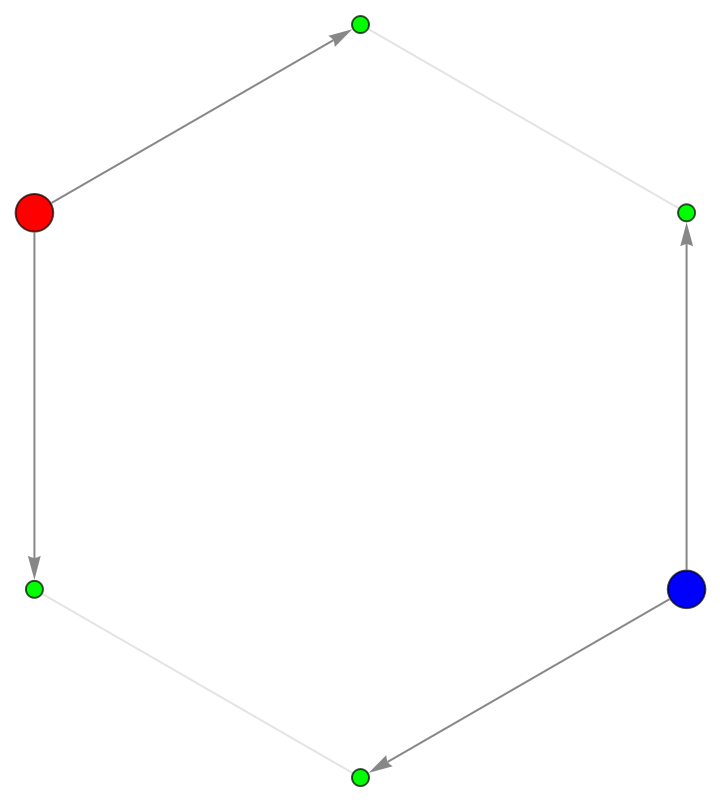Inputs with disconnected components may return only one component:

 In:=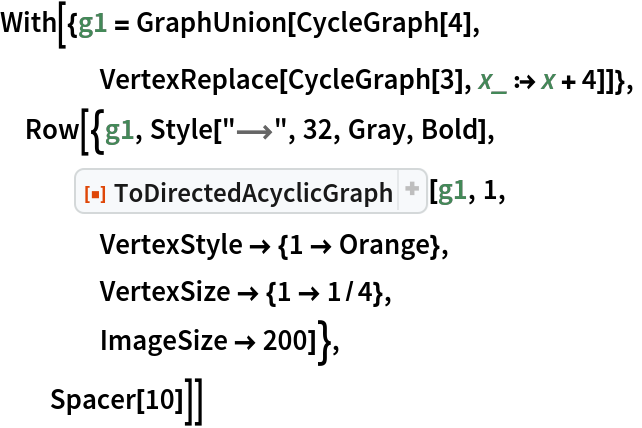Out=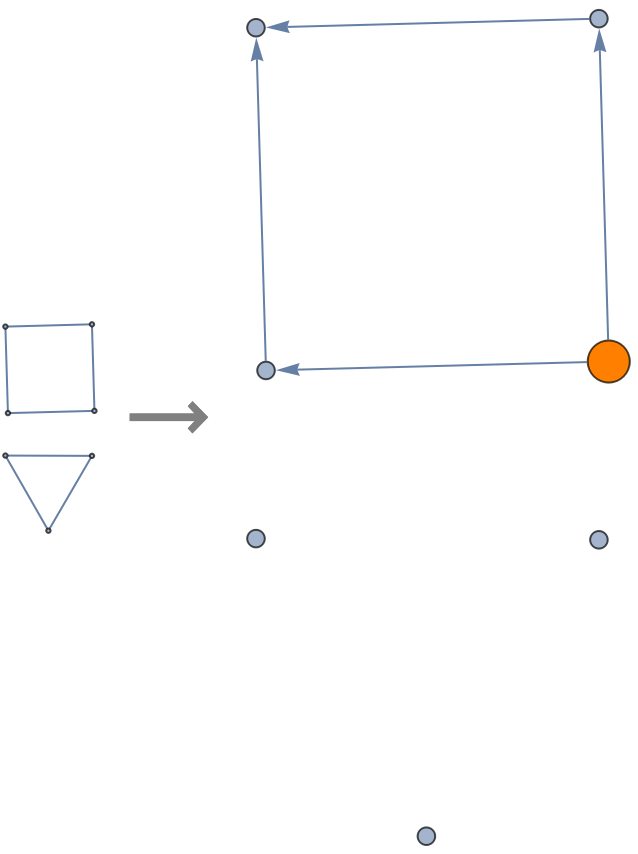This can be fixed by specifying initial vertices on each component:

 In:=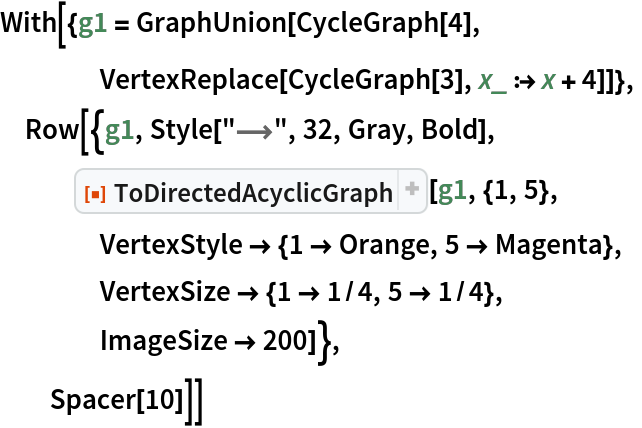Out=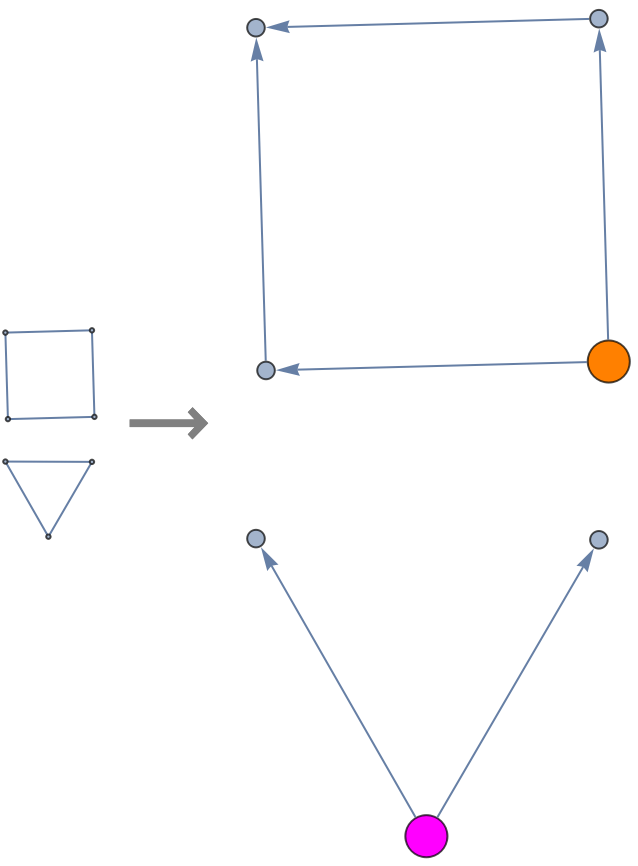The function will balk at nonsense inputs:

 In:=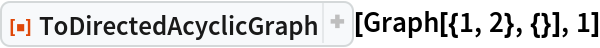Out=In:=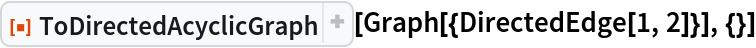Out=In:=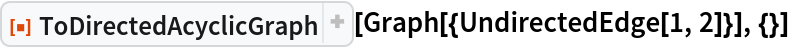Out=### Neat Examples (2)

Count possible walks on all Petersen graph outputs:

 In:=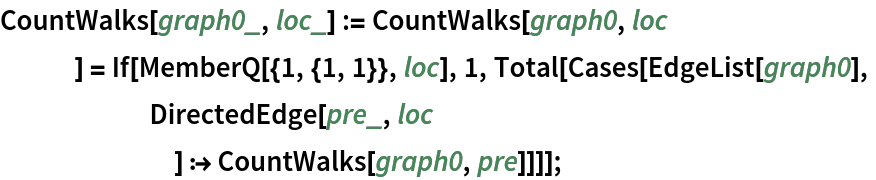In:=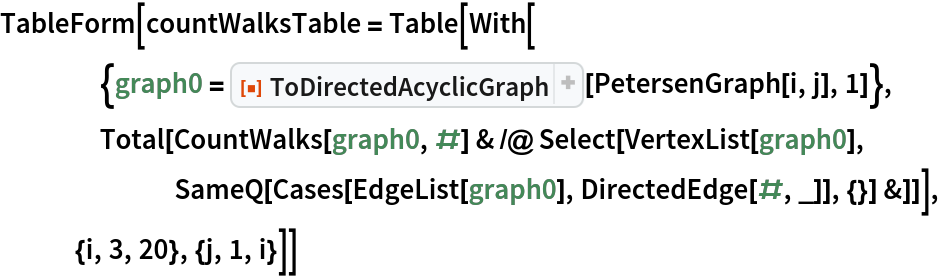Out=List a sequence that does not have an entry in the OEIS:

 In:=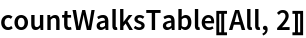Out=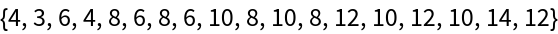Plot the possibly new sequence:

 In:=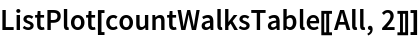Out=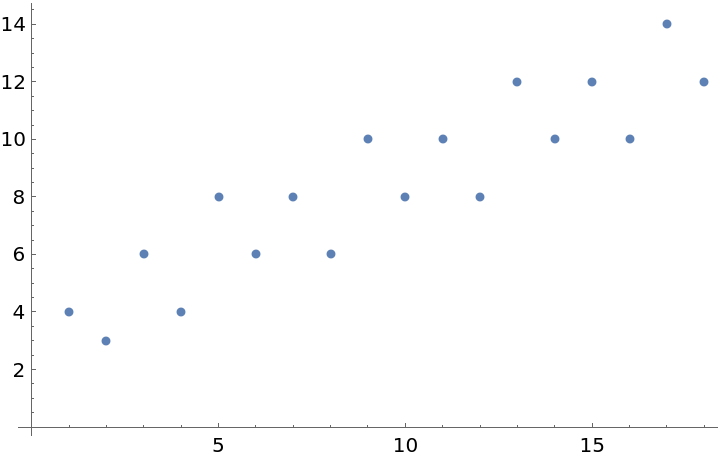Conjecture the form of a linear recurrence:

 In:=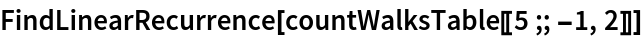Out=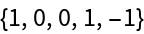Compare with depictions to try and formulate a proof argument:

 In:=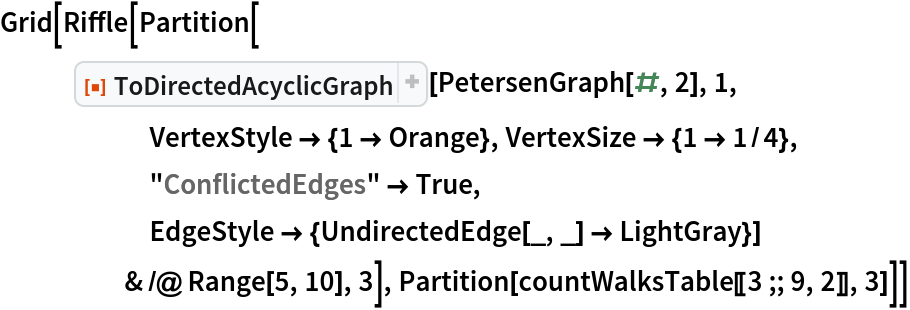Out=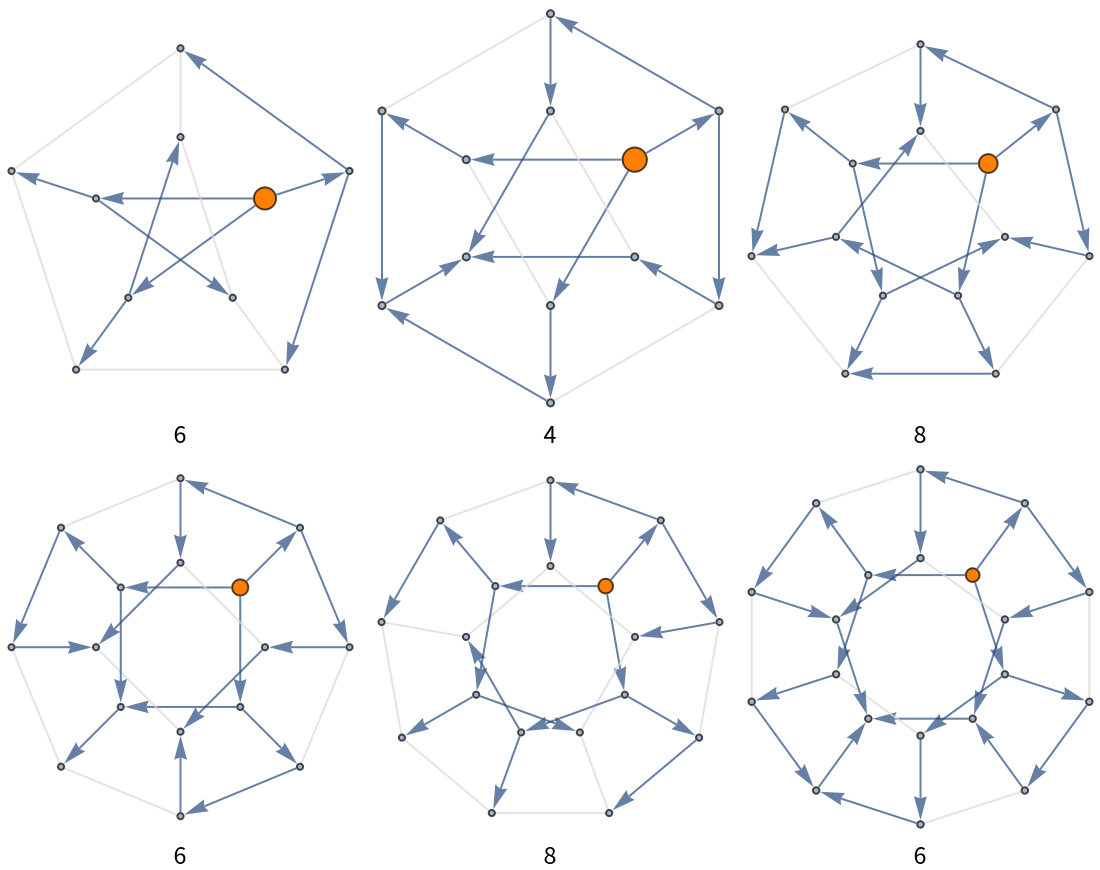Resolve conflicted edges in a square grid:

 In:=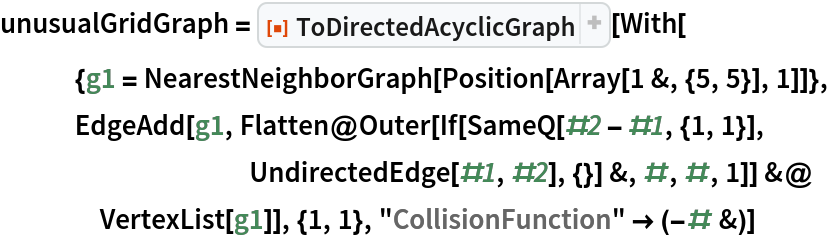Out=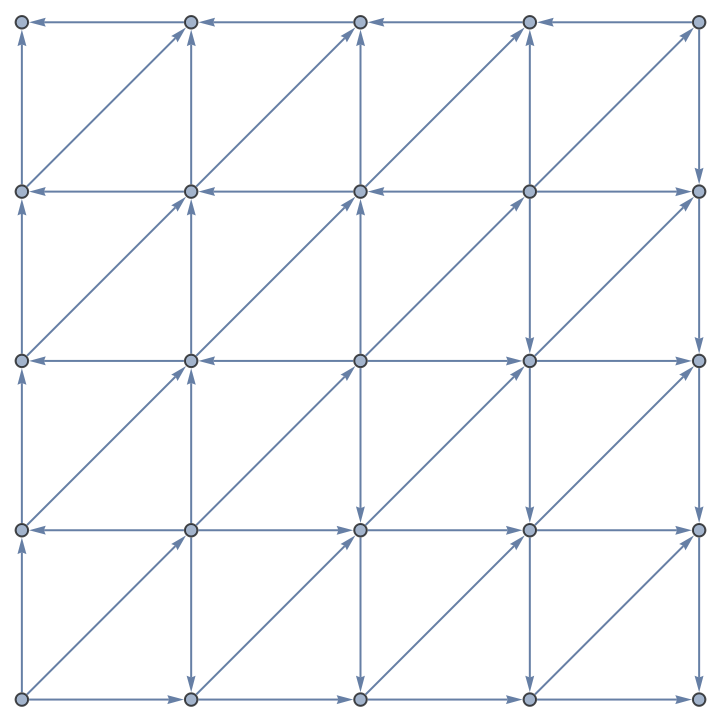Calculate part of a numerical triangle associated with the Schroeder numbers (cf. OEIS A033877):

 In:=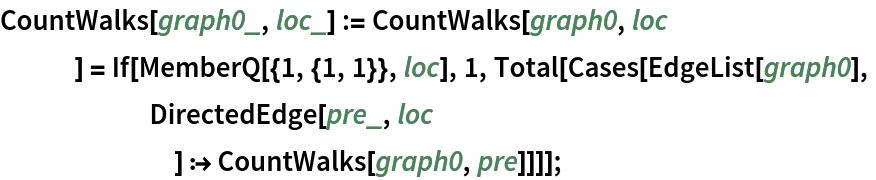In:=Out=